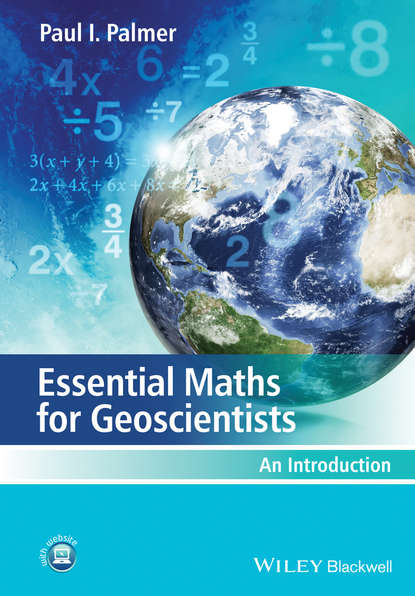﻿ Essential Maths for Geoscientists. An Introduction (Paul I. Palmer) – скачать в fb2, epub, pdf на FenzinСкачать полностью

# Essential Maths for Geoscientists. An Introduction

Электронная книга
Добавлено 21.02.2020
Год издания: 2018
Аннотация

Maths for Geoscientists is an accessible, student-friendly introduction to the essential mathematics required by those students taking degree courses within the Geosciences. Clearly structured throughout, this book carefully guides the student step by step through the mathematics they will encounter and will provide numerous applied examples throughout to enhance students understanding and to place each technique into context. Opening with a chapter explaining the need for studying mathematics within geosciences the book then moves on to cover algebra, equations, solutions, logarithms and exponentials, statistics and probability, trigonometry, vectors and calculus. The final chapter helps to bring it all together and provides the students with sample projects to test their knowledge. Worked applied examples are included in each chapter along with applied problem questions which are a mix of straightforward maths questions, word questions (developing maths to words), and more involved questions that involve the manipulation and interpretation of real and synthetic data.

Полная версия
﻿
Отзывы о книге Essential Maths for Geoscientists. An Introduction
Нет отзывов
Добавить отзыв

Чтобы добавить отзыв Вам нужно зарегистрироваться или авторизироваться